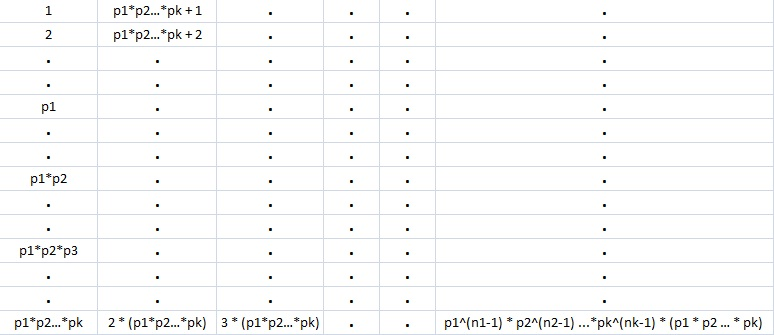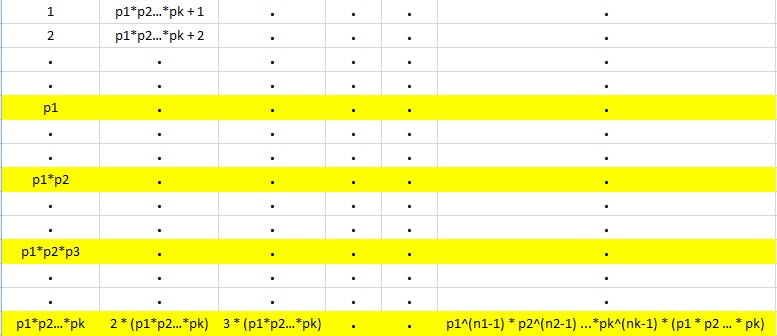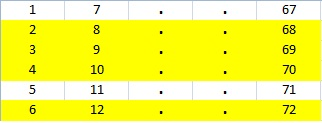Proof of Euler's Totient Function

 Part 1 Part 2

Theorem :
The number of numbers co-prime to a given number, say n, is denoted by phi(n).
If n = p1^n1 * p2^n2 * p3^n3 ... pk^nk
then phi(n) = phi(p1^n1 * p2^n2 * p3^n3 ... pk^nk)
= p1^(n1-1) * p2^(n2-1) -- pk^(nk-1) * phi(p1 * p2 * p3 ... pk)
where p1,p2,p3...pk are prime factors of n.

Proof:

Consider the numbers from 1 to n arranged in an array as shown below:The last element in the column 1 is p1*p2...pk and that in column 2 is 2*(p1*p2...pk).
Correspondingly, the last element in the last column is p1^(n1-1) * p2^(n2-1)...pk^(nk-1)*(p1 * p2 -- pk) i.e. n.
Thus there are p1^(n1-1) * p2^(n2-1)...pk^(nk-1) columns.
Now we begin cancelling rows (with yellow highlight) where in the numbers p1,p2...pk,2p1,2p2...2pk,p1p2,2p1p2 etc. occur in the first column. i.e. whenever the first column contains prime factors of n or their multiplies, we eliminate the corresponding row.
The numbers remaining untouched after this process are all the numbers coprime to n.The numbers contianed in the first column must correspond to phi(p1 * p2...pk) i.e. The numbers remaining in the first column are coprime to the last element in that column i.e. p1 * p2...pk.
Obviously, the number of untouched elements in all columns is the same. Now, since there are p1^(n1-1) * p2^(n2-1)...pk^(nk-1) columns, the total number of untouched elements is p1^(n1-1) * p2^(n2-1)...pk^(nk-1) * phi(p1 * p2...pk).
Thus phi(n) = phi(p1^n1 * p2^n2...pk^nk)
= (p1^(n1-1) * p2^(n2-1) -- pk^(nk-1) * phi(p1 * p2 * p3 -- pk)
To make things clear, consider an example where n = 72
Now 72 = 9 * 8 = 3^2 * 2^3
Thus here p1 = 3 & p2 = 2.
So, our array can be constructed as shown below
Now we eliminate rows R2, R3, R4, R6 as they contain prime factors of 72 and their multiples.
Our array then transforms to:So, the number of un-removed elements in column 1 are 2 and they are all coprime to 6. Since there are 12 such columns, we have phi(72) = 12 * phi(6) = 12 * 2 = 24.
This also corresponds to phi(72) = phi(3^2 * 2^3) = 3^(2-1) * 2^(3-1) * phi(3*2) = 3^1 * 2^2 * phi(6) = 12 * phi(6)
Though this proof works out the final result by using an example, I think the theorem can also be proved by using sets and/or by using remainder theorem.
Haven't gotten to it yet. The 2nd part of the proof, where we further simplify phi(p1*p2*...*pk) can be found at the bottom portion of this page Discovering Euler's Totient Function

 Quick Comment : * Name Email ( Your email id is safe with us! ) * Comments * Type the number of characters in the word "HUMAN"